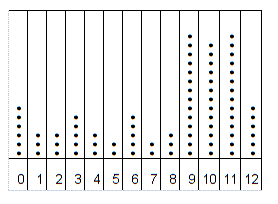# Center and Variation of Data

Given a numerical data distribution, we can answer questions about the central tendency of the data and its variation.

We can use different measures like mean, median, or mode to represent the center of the data with a single number.

The variation can also be expressed with a single number, most simply by finding the range , or difference between the highest and lowest values. It can also be expressed using the standard deviation or variance .

For example, in the dot plot below, most of the data is clustered in the range $9-11$ . The modes are $9$ and $11$ , the median is $9$ , and the mean is about $8.2$ .

The range is $12$ , the variance is about $14.4$ , and the standard deviation is about $3.8$ .Another way to express the central tendency and variation of a numerical data set is with a box-and-whisker plot .